﻿

### On the extrinsic principal directions of Riemannian submanifolds

#### Abstract

The Casorati curvature of a submanifold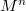$M^n$ of a Riemannianmanifold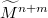${\widetilde{M}^{n + m}}$ is known to be the normalized square of the lengthof the second fundamental form,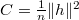$C = \frac{1}{n}\|h\|^2$, i.e., inparticular, for hypersurfaces,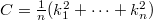$C = \frac{1}{n}(k_1^2 + \dots +k_n^2)$, whereby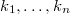$k_1,\dots,k_n$ are the principal normalcurvatures of these hypersurfaces. In this paper we in additiondefine the Casorati curvature of a submanifold$M^n$ in aRiemannian manifold${\widetilde{M}^{n + m}}$ at any point$p$ of$M^n$ in any tangentdirection$u$ of$M^n$. The principal extrinsic (Casorati)directions of a submanifold at a point are defined as an extensionof the principal directions of a hypersurface$M^n$ at a point in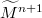${\widetilde{M}^{n + 1}}$. A geometrical interpretation of the Casorati curvature of$M^n$ in${\widetilde{M}^{n + m}}$ at$p$ in the direction$u$ is given. Acharacterization of normally flat submanifolds in Euclidean spacesis given in terms of a relation between the Casorati curvaturesand the normal curvatures of these submanifolds.

DOI Code: 10.1285/i15900932v29n2p41

Keywords:
Casorati curvature; principal direction; normal curvature; squared length of the second fundamental form

Full Text: PDF